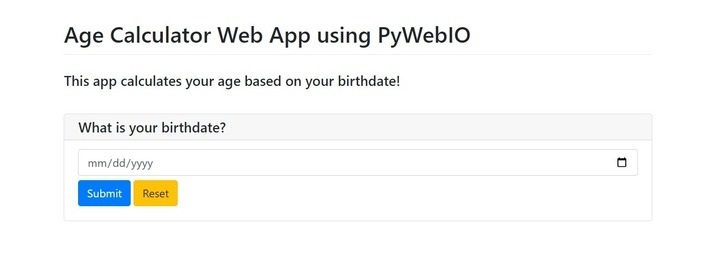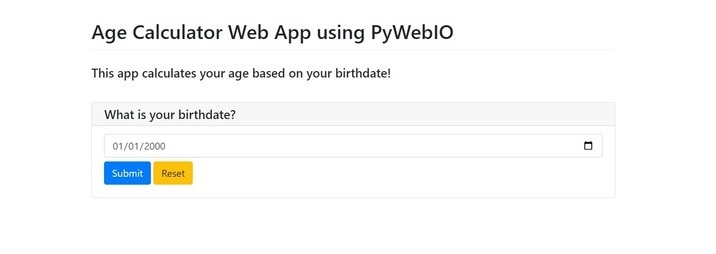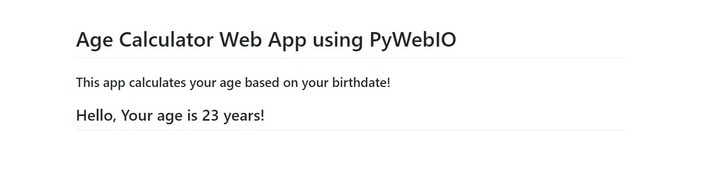# How to create AGE Calculator Web App PyWebIO in Python?

Those who wish to practice their Python skills and learn how to develop a small web app can quickly and amusingly create an age calculator web app using PyWebIO in Python. Interactive online apps are simple to construct thanks to the Python library PyWebIO. This project's online age calculator uses PyWebIO to determine a user's age based on their birthdate.

To calculate dates for this web application, we'll use the datetime package that comes with Python by default. The software requires the user's name and birthdate, which then calculates their age in years using the current date. The output will be shown on the web page using PyWebIO's output routines.

The Age Calculator Web App is created by installing the PyWebIO library, importing the necessary modules, defining the main function to compute the age, starting the server to run the app, and finally running the script and accessing the app on a web browser.

## Steps to Create AGE Calculator Web App PyWebIO

• Step 1 − Install PyWebIO: The PyWebIO library must be installed first using pip. To accomplish this, run the following command at the terminal or command line −

pip and install pywebio

• Step 2 − Import the required modules: After installing the PyWebIO library, the following action is to import the required modules. We must import the datetime module and the input, output, and start server methods from the PyWebIO library to execute date calculations.

from pywebio.input import *
from pywebio.session import *
from pywebio.output import *
from datetime import datetime

• Step 3 − Provide the primary function to determine the user's age depending on their birthdate. The third step is to specify the main function that will do this. Using the user's birthdate as input, this method will utilize the datetime module to determine the user's age in years. Using PyWebIO's output functions, the outcome will be output to the web page.

• Step 4 − Launch the server so the app may run: The next step is to launch the server so that the app can execute after the main function has been established. The PyWebIO start server function can be used to accomplish this. The primary function and the server port number are the two inputs this function accepts.

• Step 5 − To utilize the app, run the script and navigate to http://localhost in your web browser: The script containing the main function must be executed, and the server must be started before the app may be used. Once the server is up and running, we can view the Age Calculator Web App by navigating to http://localhost in our web browser.

### Example

In this example, we import the appropriate modules, including datetime, for working with dates and many PyWebIO library functions, such as input, output, and start_server.

We specify the age calculator's main function. This function takes input before calculating their age in years using the datetime module. Lastly, the output functions of PyWebIO are used to display the outcome on the web page.

Using PyWebIO's start server function, we launch the server to run the program in the if main block. This function accepts two arguments: the primary function (in this case, the age calculator) and the port number that the server should use (we chose 80 for simplicity). The start server function invokes the age calculator function, which launches the server on port 80 when the script is executed.

from datetime import datetime
from pywebio.input import *
from pywebio.output import *
from pywebio import start_server

def age_calculator():
put_markdown('# Age Calculator Web App using PyWebIO')
birth_date = input("What is your birthdate?", type=DATE)
birth_date = datetime.strptime(birth_date, "%Y-%m-%d")
current_date = datetime.now()
age_in_years = current_date.year - birth_date.year - ((current_date.month, current_date.day) < (birth_date.month, birth_date.day))
put_markdown("## Hello, Your age is %d years!" % (age_in_years))

if __name__ == '__main__':
start_server(age_calculator, port=80)


### OutputWe only need to open our web browser and navigate to http://localhost to use the app. We can enter the birthdate into the software available at this URL to determine the age.Enter your date of birth and click on "Submit" button −## Conclusion

In conclusion, this code develops a straightforward web application that uses PyWebIO and Python to determine a user's age based on their birthdate. It shows how to use PyWebIO to build a simple web application and how to use the datetime module to compute dates.

Updated on: 11-May-2023

169 Views